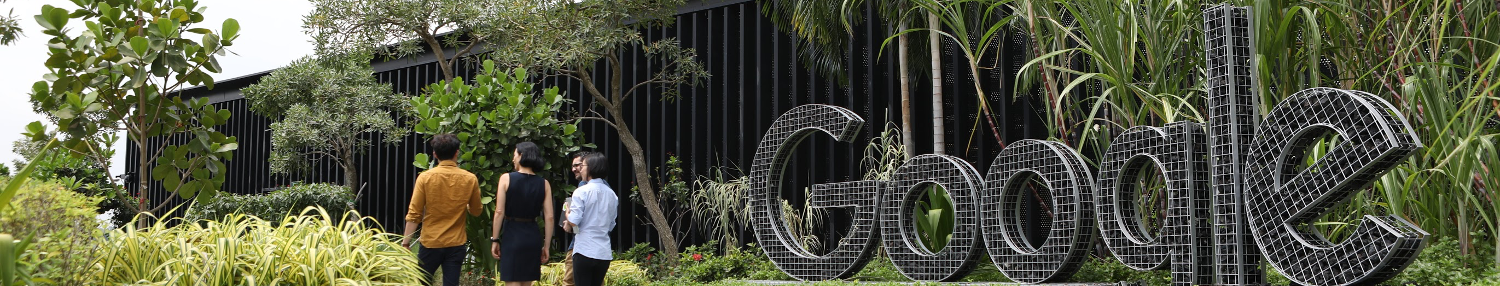-

# How to measure the hight of the building using Barometer

## Respuestas de entrevistas

3 respuestas

1

use speed of sound calculaiton, physics formaula force distance mass, etc

Anónimo en

1

Barometer measures presssure. At low altitude above sea level pressure drops by 1.2 kPa for every 100 meters. Measure pressure at base of build. Then at top of building. Based on the difference in pressure figure the height of buiding

Anónimo en

0

Drop the barometer from the top of the building and note the time it takes to fall on the ground. Now acceleration = g = 9.8 u= 0 s = 1/2 g(t*t) Assuming, if the time it takes is 5 seconds s= 1/2*9.8*25 = 122.5 mtrs.

Kashif en